# Marginal Costing – Corporate and Management Accounting MCQ

1

Marginal Costing – Corporate and Management Accounting MCQ

1 / 68

: P/V Ratio is 25% and margin of safety is ₹ 6,00,000, the amount of profit is:

2 / 68

The following information is given: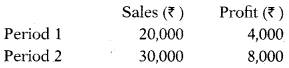Sales to earn a profit of ₹ 16,000 will be:

3 / 68

June 2019: The effect of sale price reduction always reduces the P/V ratio to raise and shorten the

4 / 68

June 2019: Selling price per unit ₹ 20, Trade discount 5% of selling price, cash discount 2% on sales, Material cost ₹ 3, Labour cost ₹ 4, Fixed overheads ₹ 22,000 and variable overheads 80% of labour cost. what would be the net profit if sales are 10% above the BEP?

5 / 68

: The total cost and profit during two periods are as follows: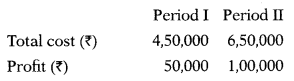The profit volume ratio will be:

6 / 68

Selling price ₹ 20 per unit, Variable cost ₹ 15 per unit and Fixed cost ₹ 48,000. What will be BEP sales (in ₹ ) and Profit if actual sales are 40% more than BEP sales?

7 / 68

Dec 2018: Which of the following techniques of costing is also known as out-of-pocket costing?

8 / 68

Dec 2018: If the P/V Ratio is 30%, Margin of Safety is 40% and BEP is ₹ 48 Lakh, then the profit will be:

9 / 68

Dec 2018: The production cost of 1,0 units of an article is as follows:

 Costs ₹ Direct Material 4,00,000 Direct Wages 3,00,000 Fixed & Variable Overheads 2,00,000The company produced 5,000 units and sold at ₹ 1,000 per unit and earned a profit of ₹ 10,00,000. The amount of variable overhead per unit is:

10 / 68

Dec 2018: Given, Sales ₹ 80 Lakh; Net Profit ₹ 8 Lakh and Fixed Cost ₹ 12 Lakh. On the basis of the data, if sales is ₹ 120 Lakh, then the profit will be:

11 / 68

Dec 2018: If the standard output for 8 hours is 200 units and the actual output in 10 hours is 350 units, the efficiency level will be:

12 / 68

June 2018: ABC Ltd. shows break-even sales of ₹ 40,500 and budgeted sales of ₹ 50,000. Compute the margin of safety ratio?

13 / 68

June 2018: Actual overheads for the year ending 31st March 2017 were ₹ 21,000, whereas the overhead absorbed shows an over absorption of ₹ 1,000 for the same period. If the direct labour cost is ₹ 1,00,000, then the overhead absorption rate based on direct wages would be:

14 / 68

June 2018: When the sales increase from ₹ 40,000 to ₹ 60,000 and profit increases by ₹ 5,000, the P/V ratio is:

15 / 68

June 2018: A company, which has a margin of safety of ₹ 2,00,000 makes a profit of ₹ 40,000. If the fixed cost is ₹ 2,50,000, break-even sales of the company would be:

16 / 68

June 2018: A manufacturing company provides you with the following information for the coming month:

 Budgeted sales revenue ₹ 7,50,000 Budgeted contribution ₹ 3,00,000 Budgeted profit ₹ 75,000

17 / 68

: Which of the statement is not true in respect of cost-volume-profit analysis?

18 / 68

June 2018: If the P/V ratio of a product is 25% and the selling price is ₹ 25 per unit, the marginal cost of the product would be:

19 / 68

Dec 2017: R.V. Ltd., made a sale for ₹ 4,50,000 in the first half and for ₹ 5,00,000 in the second half of 2016. In this year the total cost for the first and the second half of the year were respectively ₹ 4,00,000 and ₹ 4,30,000. If there is no change in selling price and variable cost and that the fixed expenses are incurred equally, the break-even sales for the whole year is:

20 / 68

Dec 2017: Mr. R’s sales and profit in 2015 were respectively ₹ 1,20,000 and ₹ 8,000. His sales & profit in 2016 were ₹ 1,40,000 & ₹ 13,000 respectively. In this case his margin of Safety in 2016 was:

21 / 68

Dec 2017: When fixed costs are ₹ 90,000, the ratio of variable cost to sales is 75% and the break-even point occurs at 60% of the capacity sales, the capacity sales are:

22 / 68

Dec 2017: Marginal Costing in America is called as:

23 / 68

Consider the following statements:(1) Marginal costing and absorption costing are the same.(2) For decision-making, absorption costing is more suitable than marginal costing.(3) Cost-volume-profit relationship also denotes the break-even point.(4) Marginal costing is based on the distribution between fixed and variable costs.

24 / 68

Assertion (A):ProRt volume ratio is considered to be the best indicator of the profitability of the business.
Reason (R):If the profit volume ratio improved, it will result in better profits.

25 / 68

Which of the following is not true?

26 / 68

June 2017: Under marginal costing system, product costs are:

27 / 68

June 2017: If the total cost of producing 20,000 units of a product is ₹ 90,000 and if 25,000 units will be produced, then the total cost will be ₹ 1,05,000 and the selling price is ₹ 8 per unit. The break-even point will be:

28 / 68

June 2017: A plant is operating at 60% capacity. The fixed costs are ₹ 30,000, the variable costs are ₹ 1,00,000 and the sales amount to ₹ 1,50,000. The percentage of capacity at which the plant should operate to earn a prophet of ₹ 40,000 will be:

29 / 68

June 2017: Yadhav Co. has an annual fixed cost of ₹ 1,20,000. In 2015 sales amounted to ₹ 6,00,000 as compared to ₹ 4,50,000 in 2014 and prophet in 2015 was ₹ 50,000 higher than in 2014. If there is no need to expand the company’s capacity. The prophet or loss in 2016 on a forecasted sales of ₹ 9,00,000 will be:

30 / 68

A company sells its product at ₹ 15 per unit. In a period if it produces and sells 8,000 units, it incurs a loss of ₹ 5 per unit. If the volume is raised to 20,000 units, it earns a profit of ₹ 4 per unit. Break-even point in units will be:

31 / 68

A factory engaged in manufacturing plastic buckets is working at 40% capacity and produces 10,0 buckets per annum. The present cost-break-up for one bucket is as under:

 Materials ₹ 10 Labour ₹ 3 Overheads ₹ 5 (60% fixed)The selling price per bucket ₹ 20. If the factory operates 90% of capacity the profit will be:

32 / 68

In a period sales amount to ₹ 2,00,000, net profit ₹ 20,000 and Fixed overheads are ₹ 30,000. If sales ₹ 3,00,000 profit will be:

33 / 68

From the following data, the P/V ratio will be: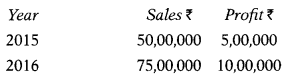34 / 68

June 2017: In a purely competitive market, 10,000 pocket transistors can be manufactured and sold and certain profit is generated. It is estimated that 2,0 pocket transistors need to be manufactured and sold in a monopoly market to earn the same profit. Profit under both conditions is targeted at ₹ 2,00,000. The variable cost per transistor is ₹ 100 and the total fixed costs are ₹ 37,000. You are required to find out the unit selling price per transistor under competitive condition.

35 / 68

June 2017: Mr Mahesh has a sum of ₹ 3,00,000 which invested in a business. He wishes for a 15% return on his fund. It is revealed from the present cost data analysis that the variable cost of operation is 60% of sales and fixed costs are ₹ 1,50,000 p.a. On the basis of this information, you are required to find out the sales volume to earn a 15% return.

36 / 68

: Ramya Ltd. furnishes the following information:Production 10,000 units,Sales 10,000 units,Selling price ₹ 12 per unit,Variable cost ₹ 6 per unit,Fixed costs ₹ 40,000 per annum (normal capacity of 10,000 units)Profit/Loss under marginal costing method will be:

37 / 68

Statement -1When there are no inventories, the profit figure under marginal costing and absorption costing is identical.
Statement – IIInventories are valued at cost of production in absorption and marginal costing systems.

38 / 68

Following information is given for a product of a manufacturing company:Material ₹ 18 per unit; other variable costs ₹ 22 per unit; and fixed expenses ₹ 18 per unit. The selling price is ₹ 75 per unit. The company is presently producing 80.0 units at 80% capacity. The company received an offer for 20,000 units from a foreign customer. The minimum price to be accepted from a foreign customer, if the company wants to earn 20% on foreign sales will be

39 / 68

In a purely competitive market, 10,000 pocket transistors can be manufactured and sold and certainprofit is generated. It is estimated that 2.0 pocket transistors need to be manufactured and sold in a monopoly market to earn the same profit. Profit under both conditions is targeted at ₹ 2,00,000. The variable cost per transistor is ₹ 100 and total fixed costs are ₹ 37,000. Unit selling price per transistor under monopoly condition will be

40 / 68

A radio manufacturer finds that while it costs 16.25 per unit to make a component, the same is available in the market at ₹ 5.75 each. Continuous supply is also fully assured. The break-up of costs per unit is as follows:Materials: ₹ 2.75Labour: ₹ 1.75Other variable expenses: ₹ 0.50Depreciation & other fixed costs: ₹ 1.25The best option for the manufacturer will be

41 / 68

June 2016: The ratio of variable cost to sales is 75%. The break-even point occurs at 64% of the capacity sales when fixed cost is ₹ 1,20,000. The 100% capacity sales will be

42 / 68

June 2016: Aman Ltd. sells its products at ₹ 16 per unit. In a period, if it produces and sells 20,000 units, it incurs a loss of ₹ 2 per unit. If the volume is doubled, it earns a profit of ₹ 2.20 per unit. The amount of fixed cost and breakeven point (in units) will be

43 / 68

June 2016: A company producing three products, viz., X, Y and Z has a sales mix in the ratio of 2:1:3. The profit volume ratio of the products X, Y and Z are 15%, 30% and 20% respectively. The total fixed cost of the company is ₹ 3,50,000. The break-even point of the company will be

44 / 68

: Cost-Volume-Profit analysis is based on several assumptions. Which one of the following is not one of these assumptions

45 / 68

Following data are given: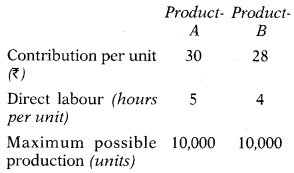Direct labour hours available are 72,000 hours.What should be the number of units of A and B to be produced to maximize the profit of the company?

46 / 68

There are two similar plants under the same management. The management desires to merge these two plants. The following particulars are available: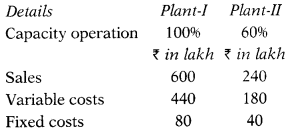The capacity of the merged plant to be operated for the purpose of break-even will be

47 / 68

Cost-volume-profit (CVP) analysis is based on several assumptions. Which one of the following is not relevant for such an analysis

48 / 68

Choose the correct statements from the following:(1) Marginal costing and absorption costing are the same(2) For decision making, absorption costing is more suitable than marginal costing(3) Cost-volume-profit relationship also denotes break-even point(4) Marginal costing is based on the distinction between fixed and variable costs.

49 / 68

Dec 2015: A company sells its product at ₹ 15 per unit. In a period, it produces and sells 8,000 units and incurs a loss of ₹ 5 per unit. If the sales volume were to be raised to 20,000 units, it could earn a profit of ₹ 4 per unit. The Break-even point (in units) will be

50 / 68

Statement-I:The margin of safety represents the difference between sales at the break-even point and total sales.
Statement-II:The margin of safety can be expressed as a percentage of total sales or in value or in terms of quantity.

51 / 68

Which of the following are advantages of marginal costing :(1) Pricing decision(2) True profit(3) Difficulty to classify(4) Ignores time value(5) Break-even analysis(6) Contribution is not final(7) Control over expenditure

52 / 68The following data is obtained from the records of Mayur Ltd:

53 / 68

Assertion (A):The business earns a surplus of sale revenue over variable costs, which is called a contribution.
Reason (R):Once fixed costs are fully recovered such excess contribution is termed as profit.Select the correct answer from the options given below

54 / 68

Dec 2015: Manoj Ltd. manufactures three products P, Q and R. The unit selling price of these products are ₹ 100, ₹ 160 and ₹ 75 respectively. The corresponding unit variable costs are ₹ 50, ₹ 80 and ₹ 30. The proportions (quantity-wise) in which these products are manufactured and sold are 20%, 30% and 50% respectively. Total fixed costs are ₹ 14,80,000. Overall breakeven quantity is

55 / 68

The following information is given about Zac Ltd. dealing in musical instruments:P/V ratio 50% Margin of safety 40%If the sales volume is ₹ 50,00,000 the net profit will be

56 / 68

Dec 2015: Z Ltd. recorded sales of ₹ 60 lakh in 2014 as compared to ₹ 45 lakh in 2013. Profit for 2014 was ₹ 5 lakh higher than that in 2013. If the annual fixed costs amount to ₹ 12 lakh, the profit on projected sales of ₹ 90 lakh will be

57 / 68

June 2015: The selling price of a product-X is ₹ 50 per unit, variable cost ₹ 20 per unit and 2 kg of raw material are needed to produce a unit of product-X. The contribution per kg of raw material will be –

58 / 68

June 2015: The selling price of a product is 1550 per unit, variable cost ₹ 50 per unit and fixed cost ₹ 10,000. The number of units required to be sold to earn a profit of ₹ 10,000 will be –

59 / 68

A product is sold at ₹ 150 per unit and its variable cost is ₹ 70 per unit. The fixed expenses of the business are ₹ 8,000 per year. The Break-even point (in units) is

60 / 68

When the sales increase from ₹ 45,000 to ₹ 60,000, the profit increases by ₹ 5,000. P/V Ratio would be

61 / 68

June 2015: A company has an annual fixed cost of ₹ 1,68,000. In the year 2013-2014, sales amounted to ₹ 6,00,000 as compared to ₹ 4,50,000in the preceding year 2012-2013. The profit in the year 2013-2014 was ₹ 42,000 more than that in the year 2012-2013. The break-even sales of the company are

62 / 68

What is the margin of safety, if profit is equal to 140,000 and P/V ratio is 25%

63 / 68

Dec 2014: A company that has a margin of safety of ₹ 4,00,000 makes a profit of ₹ 1,00,000. If its fixed cost is ₹ 5,00,000, then break-even sales are:

64 / 68

The following information relates to a product:Direct materials: 10 kg @ ₹ 0.50 per kg.Direct labour: 1 hour 30 minutes @ ₹ 4 per hourVariable overheads: 1 hour 30 minutes @ ₹ 1 per hourFixed overheads @ ₹ 2 per hour (based on a budgeted production volume of 90,0 direct labour hours for the year)Selling price per unit: ₹ 17 The break-even point is

65 / 68

Which of the following formula cannot be used for calculating the contribution

66 / 68

Dec2014: For a given product, the selling price per unit is 15, the variable cost per and units sold during the period are 35,000. The margin of safety is

67 / 68

Dec 2014: For a given product, the sales of a company @ ₹ 200 per unit is ₹ 20,00,000. Variable cost is ₹ 12,00,000 and fixed cost is ₹ 6,00,000. The capacity of the factory is 15,000 units. Capacity utilization at break-even point level

68 / 68

: Which of the following formula cannot be used for calculating the P/V ratio

This site uses Akismet to reduce spam. Learn how your comment data is processed.